## Numerical integration and differentiation

### Quick description

Given a function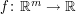, compute (approximately) the definite integral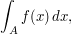for some subset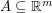or to compute (approximately) the a derivatives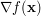, or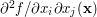etc., using only a finite (or small) number of function evaluations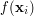,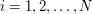.

The most efficient numerical integration methods have been developed for scalar problems (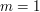), including methods such as Gauss quadrature and Romberg integration. But there are good methods for many regions in higher dimensions. Regions with high dimensionality (e.g.,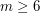) are a particular challenge, as are integrands with singularities or localized "spikes".

### Prerequisites

Calculus, interpolation.

### Example 1: Integrate (or differentiate) the interpolant

If you have an effective and accurate method of interpolation, you can use that to construct an integration method as the integral of the interpolant.

### Example 2: If you have a singularity that has an simple form, use product integration methods

For example, for computing integrals of the form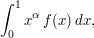where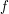is smooth on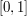, determine a polynomial interpolant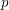of, and compute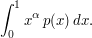This technique is also useful for dealing with smooth functions with localized "spikes" that would otherwise require very many integration points, even with adaptive methods: for example, consider computing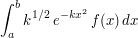with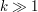. By using an interpolant ofon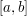and computing the resulting integral exactly, the only errors incurred are due to the interpolation of, not the fact that the integral has a "spike".

### More examples

Some examples for multidimensional integration would be nice to see here.

### To find the nth derivitive of f(x):

Use any value δ, which can be made small enough to approximate the derivitive to an arbitrary number of decimal places.
f'(x)≃(f(x+δ)-f(x))/δ
f''(x) ((f(x+2δ)-f(x+δ))-(f(x+δ)-f(x)))/δ=f(x+2δ)-2f(x+δ)+f(x)
f'''(x) (f(x+3δ)-f(x+2δ)+f(x+δ)-f(x))/δ
The pattern continues. The nth derivitive is
....................n
...................⎲
f(x+nδ)/δ+ ⎳ n(-1)^k f(x+kδ)/δ
...................k=1

The dots are there because the computer won't show spaces properly.

### Angela, I think you missed

Angela, I think you missed some coefficients, correct expression should be,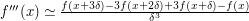And I'm sorry but its really difficult to understand the formula you write for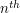derivative. I think the expression you wanted to write is the following-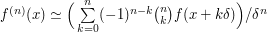## Post new comment

(Note: commenting is not possible on this snapshot.)

Before posting from this form, please consider whether it would be more appropriate to make an inline comment using the Turn commenting on link near the bottom of the window. (Simply click the link, move the cursor over the article, and click on the piece of text on which you want to comment.)